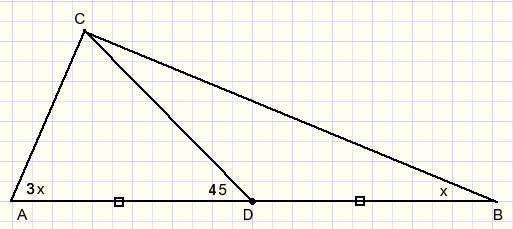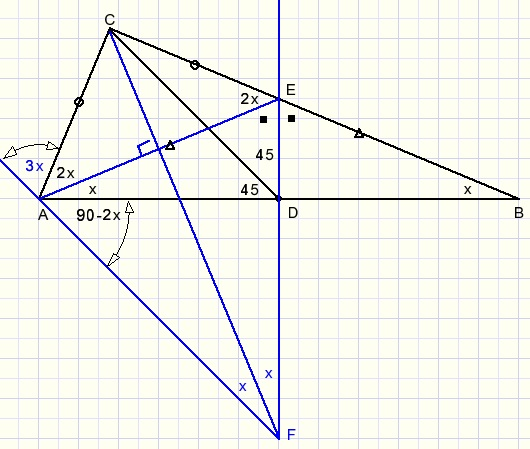geometry problem(9)Geometry problem below was found in Facebook group "PI love math".Question: prove that x=22.5

The proofstep 1:
draw perpendicular bisector of AB. E is intersection with BC.
draw AE.
LDAE=LDBE=x
LAEC=x+x=2x.....(exterior angle of ΔABE)
LCAE=2x
Conclusion: AC=EC

step 2:
draw perpendicular bisector of AE, F is intersection with extended DE.
draw FA.
LAEF=90-x
LEFC=LAFC=x
LDAF=90-2x

step 3:
x=22.50OneStat